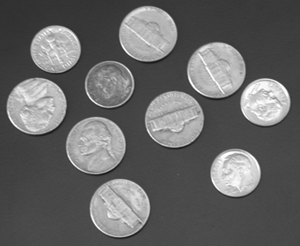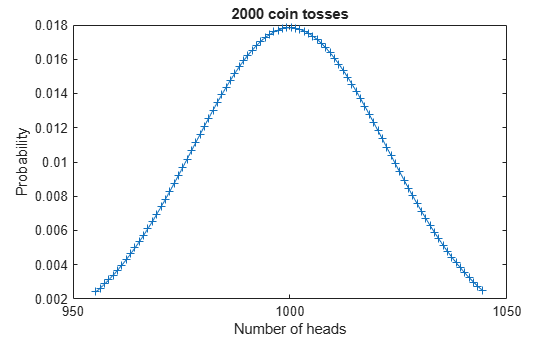# Compute Binomial Coefficients Exactly

This example shows how to get precise values for binomial coefficients and find probabilities in coin-tossing experiments using the Symbolic Math Toolbox™.Define the symbolic function, `P(n,k)`, that computes the probability for the heads to come up exactly `k` times out of `n` tosses.

```syms P(n,k) P(n,k) = nchoosek(n,k)/2^n```
```P(n, k) =  $\frac{\left(\genfrac{}{}{0}{}{n}{k}\right)}{{2}^{n}}$```

Suppose you toss a coin 2000 times. The probability that heads comes up in half of the tosses is `P(n, n/2)`, where `n = 2000`. The result is a rational expression with large numbers in both the numerator and denominator. Symbolic Math Toolbox returns the exact result.

```n = 2000; central = P(n, n/2)```
```central =  $\frac{32002369171710776786486914109075391318694138874709328653443478713621065409407558684827078034103284471897886973749969120193991903093820699875281992965144712165047076509911260111695492561758070504338841970553054102523110527916947524131958377752151574039949033529629698641642258743674137482616594745112267898723211120807230664634964544481708164904539022478043973711723559968194432939075806832004291061158908846856838256381803348565279054224647900810699299168867139792956844223922159175515314010713665400039460923455955238336479045124093228963670382989157970254873535474101598330561111077761468187361705}{1793954211366022694113801876840128100034871409513586250746316776290259783425578615401030447369541046747571819748417910583511123376348523955353017744010395602173906080395504375010762174191250701116076984219741972574712741619474818186676828531882286780795390571221287481389759837587864244524002565968286448146002639202882164150037179450123657170327105882819203167448541028601906377066191895183769810676831353109303069033234715310287563158747705988305326397404720186258671215368588625611876280581509852855552819149745718992630449787803625851701801184123166018366180137512856918294030710215034138299203584}$```

Approximate this rational number with 10-digit accuracy using `digits` and `vpa`.

```previous_digits = digits(10); vpa(central)```
`ans = $0.01783901115$`

Compute the probability that the number of "heads" differs from the expected value by no more than two standard deviations.

```sigma = sqrt(n/4); withinTwoSigma = symsum(P(n, k), k, ceil(n/2 - 2*sigma), floor(n/2 + 2*sigma))```
```withinTwoSigma =  $\frac{13683524663950565202894406832034749167239195904700934509667499858152527897032069211850781661943643686282416047513902767700103665665029054355840533069770903613738888071669349116690583596172679994408914739469288147903344743192436900584208366363134639060412272154615888058996878504469159837490119929044635173623899567959606687166441101852938440464197469845620102647004097663706638689504581437246132580182642373508676411344773424174011370403072385575195256474333714117110163049724001591393837741984544130975094551036065813178506167596616648110323149974640910169291651187202789267792477594694973714115343465}{14351633690928181552910415014721024800278971276108690005970534210322078267404628923208243578956328373980574557987343284668088987010788191642824141952083164817391248643164035000086097393530005608928615873757935780597701932955798545493414628255058294246363124569770299851118078700702913956192020527746291585168021113623057313200297435600989257362616847062553625339588328228815251016529535161470158485414650824874424552265877722482300505269981647906442611179237761490069369722948709004895010244652078822844422553197965751941043598302429006813614409472985328146929441100102855346352245681720273106393628672}$```

Approximate the result with a floating-point number.

`vpa(withinTwoSigma)`
`ans = $0.9534471795$`

Compare this result with the following two estimates derived from the cumulative distribution function (cdf) of the normal distribution. It turns out that taking the midpoint between the first integer outside and the first integer inside the two-sigma interval gives a more precise result than using the two-sigma interval itself.

```syms cdf(x) cdf(x) = 1/2 * (1 + erf((x - n/2)/sqrt(sym(n/2))))```
```cdf(x) =  $\frac{\mathrm{erf}\left(\frac{\sqrt{10} \left(x-1000\right)}{100}\right)}{2}+\frac{1}{2}$```
`estimate1 = vpa(cdf(n/2 + 2*sigma) - cdf(n/2 - 2*sigma))`
`estimate1 = $0.9544997361$`
```estimate2 = vpa(cdf(floor(n/2 + 2*sigma) + 1/2) - ... cdf(ceil(n/2 - 2*sigma) - 1/2))```
`estimate2 = $0.9534201342$`
`error1 = vpa(estimate1 - withinTwoSigma)`
`error1 = $0.001052556595$`
`error2 = vpa(estimate2 - withinTwoSigma)`
`error2 = $-0.00002704525904$`

Restore the default precision for floating-point computations.

`digits(previous_digits);`

Plot this distribution for k within the $2\sigma$-interval. The plot is a Gaussian curve.

```k = n/2 + (-2*sigma:2*sigma); plot(k, P(n,k), '-+'); title('2000 coin tosses'); xlabel('Number of heads'); ylabel('Probability');```